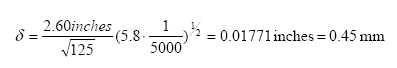# Eddy Currents

Who is Eddy, and what is he doing in our coilgun?

An inhibiting factor in coilguns is the effect of eddy currents. We did not analyze it in the Mark 4 model; instead we attempted to minimize the effects as we built it.

This page offers a brief overview of eddy currents, along with some math to verify that the iron laminations are sufficiently thin.

### Eddy Currents

The large and rapid flux changes will induce surface currents in the conductive projectile. Eddy currents always act against the applied magnetic field, reducing the absorbed kinetic energy.

Eddy currents are an important effect; a much earlier coilgun found a 24% reduction in velocity due to eddy currents in a brass firing tube. Since then, we have only used non-conductive firing tubes such as the plastic ones in this coilgun.

The solution of many eddy-current problems turns out to involve a parameter called the “skin depth”, which is:where ø = the wavelength in free space r = the material resistivity µ = the magnetic permeability of the material c = the speed of light

In mixed, but more convenient US engineering units:where f = frequency (hertz) v/vc = material resistivity, relative to copper µr = relative permeability of the material, compared to free space

The skin depth represents the distance into a material that an electromagnetic wave would penetrate. For thin or laminated iron, a useful engineering approximation to the true solution is to note that, if the width is less than two skin depths, the eddy currents on opposite sides interfere with each other, and so do not oppose the flux change.

A very rough estimate of “skin depth” in a coilgun can be made as follows. We will use frequency f = 125 Hz, which corresponds to a sine wave half-cycle of 4 ms. We assume a nail’s relative resistivity is 5.8. Also we assume relative permeability Ur = 5000.The laminated iron for this coilgun is 0.3 mm thick, so we expect it to be unaffected. However, the thinnest nail is slightly more than 1.1 mm thick and so is very likely to lose performance due to eddy currents.

In the future, we hope to test slotted or laminated projectiles that will reduce eddy currents.

 < Previous Page 35 of 37 Next >